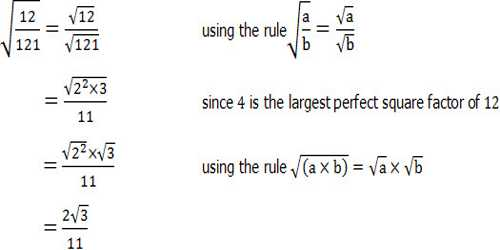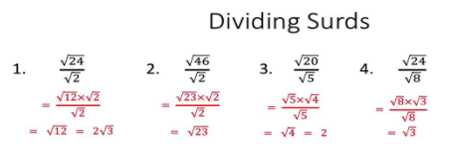Mathematic

# Division of SurdsDefinitions of surds: A root of a positive real quantity is called a surd if its value cannot be exactly determined. It is a number that can’t be simplified to remove a square root (or cube root etc). For example, each of the quantities √3, ∛7, ∜19, (16)^2/5 etc. is a surd.

More Examples:

• √2 (square root of 2) can’t be simplified further so it is a surd
• √4 (square root of 4) CAN be simplified to 2, so it is NOT a surd.

Division of Surds

In the division of surds, we need to divide a given surd by another surd the quotient is first expressed as a fraction. Then by rationalizing the denominator, the required quotient is obtained with a rational denominator. For this, the numerator and the denominator are multiplied by an appropriate rationalizing factor. In rationalization of surds, the multiplying surd-factor is called the rationalizing factor of the given surd.1. Divide: √x by √y

Solution:

√x by √y

= √x ÷ √y

= √x/√y

= √(x/y)

1. Divide the first surd by the second surd: √32, √8

Solution:

√32 divided by √8

= √32 ÷ √8

= √(32/8)

= √4 = 2.

1. Find the quotient dividing the surd √96 by the surd √16.

Solution:

Required quotient

= √96 ÷ √16

= √(96/16) = √6.

Brief explanation:

4,9,16,29,36,49,64,81,100, are often referred to as perfect squares because they give a whole number when you find their square root. Now let’s simplify the square root of 72.Here we need to think of the products that give 72, where one of the numbers is a perfect square.

What are the products that give 72? 6 times 12, 9 times 8, and 2 times 36. Can you see that two of the pairs of factors have a number which is a perfect square? What are they? Did you say 9 and 36 are perfect squares?

We’ll simplify the square root of 72 using both pairs of factors, and see which is quicker. We can say the square root of 72 equals the square root of 9 times 8, which gives the square root of 9 times the square root of 8. This equals three times root eight, which is three root eight.

You might recognize that we can simplify three root eight still further, by saying root eight equals root four times root two, which equals two root two. Now we can say, three root eight equals three times two root two, which is six root two.

So, the square root of 72 equals 6 root 2. Let’s now look at the square root of 72 as the square root of 36 times 2, which gives root 36 times root 2. This equals six times root two, or six root two.

Information Source: